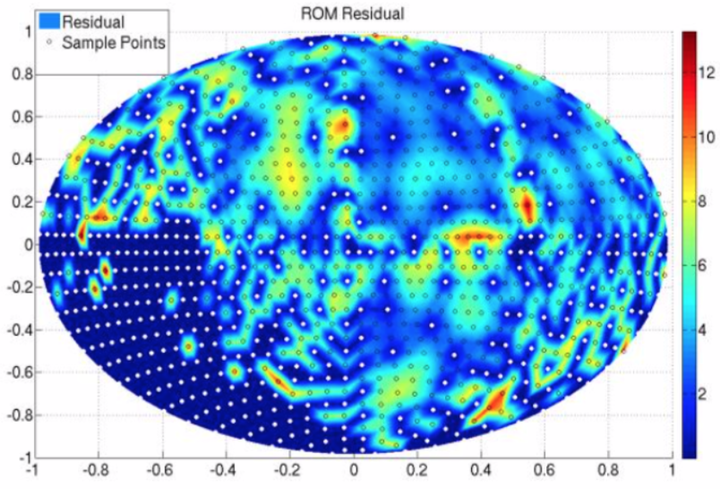# Reduced order modeling applied to the discrete ordinates method for radiation heat transfer in participating mediaDistribution of sample points generated through greedy search algorithm.

### Abstract

Radiation heat transfer is an important phenomenon in many physical systems of practical interest. When participating media is important, the radiative transfer equation (RTE) must be solved for the radiative intensity as a function of location, time, direction, and wavelength. In many heat transfer applications, a quasi-steady assumption is valid. The dependence on wavelength is often treated through a weighted sum of gray gases type approach. The discrete ordinates method is the most common method for approximating the angular dependence. In the discrete ordinates method, the intensity is solved exactly for a finite number of discrete directions, and integrals over the angular space are accomplished through a quadrature rule. In this work, a projection-based model reduction approach is applied to the discrete ordinates method. A small number or ordinate directions are used to construct the reduced basis. The reduced model is then queried at the quadrature points for a high order quadrature in order to inexpensively approximate this highly accurate solution. This results in a much more accurate solution than can be achieved by the low-order quadrature alone. One-, two-, and three-dimensional test problems are presented.

Publication
HT 2016-7010, ASME 2016 Summer Heat Transfer Conference, Washington, DC, July 10–14, 2016